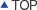MS1020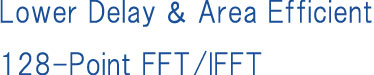MS1020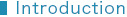The MS1020 is a Fast Fourier Transform (FFT) IP core, which computes the forward FFT and the inverse FFT (IFFT) of 128-point. The FFT is best used for applications of high speed communications like UWB (Ultra Wide Band) for ASIC hardware. Mathematec realizes Lower Delay & Area Efficiency using our original LSI circuit design technology, SPINOR. Also, the performance has been verified by the actual LSI chip.
Additionally, we take the design change request on the IP core to meet the specification of our customer's product.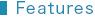– Dynamic selection between FFT and IFFT
– Continuous real-time operations of FFT frames
– 4-word length input, 8-word length output
– Operation throughput   4 points/clock
– Supports 480 Mbps operation
– Latency (means total clock cycles from first signal input to first signal output)
>> 77 clocks (out-of-order output), 108 clocks (in-order output)
– Scale factor   1/2
– Should use 2-port memory (register file memory)   Note) This core does not include memories.
- 32-bit x 32 address x 1 memory page
- 16-bit x 32 address x 4 memory pages
- 16-bit x 8 address x 4 memory pages
- 16-bit x 32 address x 4 memory pages (added in case of in-order output)
– Precision   Average SNR based on errors between this core and a Double precision FFT is
approximately 29.1dB.
Note) Average SNR on a 7-bit internal precision FFT is approximately 27.2dB.
– Deliverables
>> Synthesizable Verilog RTL source code   (Logic Synthesis is confirmed as shown in Figure 2)
>> Comprehensive Verification test bench and its vectors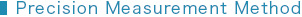FFT is calculated with double precision for the 128-point complex data of which both of Real-part and Imaginary-part are integers between -7 and +7. The outputs are divided by 2 and are rounded to the integer between -127 and +127. The result is { fk } k=0,1,–––,127.   Given the C/C++ Model of this FFT for the same complex data is { fk } k=0,1,–––,127.   We define SNR (dB) by the following formula.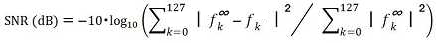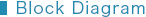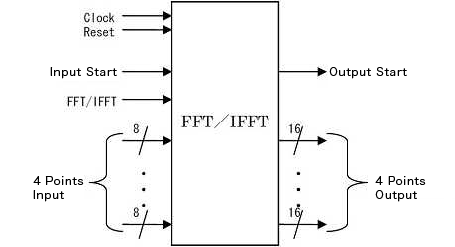Figure 1   Block Diagram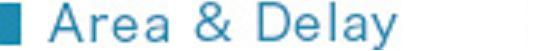The size of this IP core is depended on a Delay Constraint shown as Figure 2, and our customer can choose any one of them.

Tools
Synopsys's Design Compiler
Version   A-2007.12-SP2
Library ARTISAN   TSMC 90nm Gen.

Logic Synthesis Constraints
1. Delay Constraints   See Figure 2
2. Maximum Fanout Constraints   non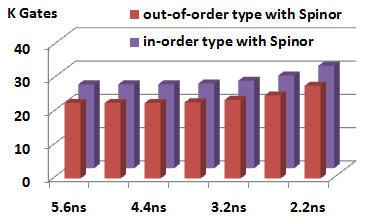Figure 2   Gate Counts & Delay Note) Figure 2 shows the 2-input-NAND-equivalent gate counts.